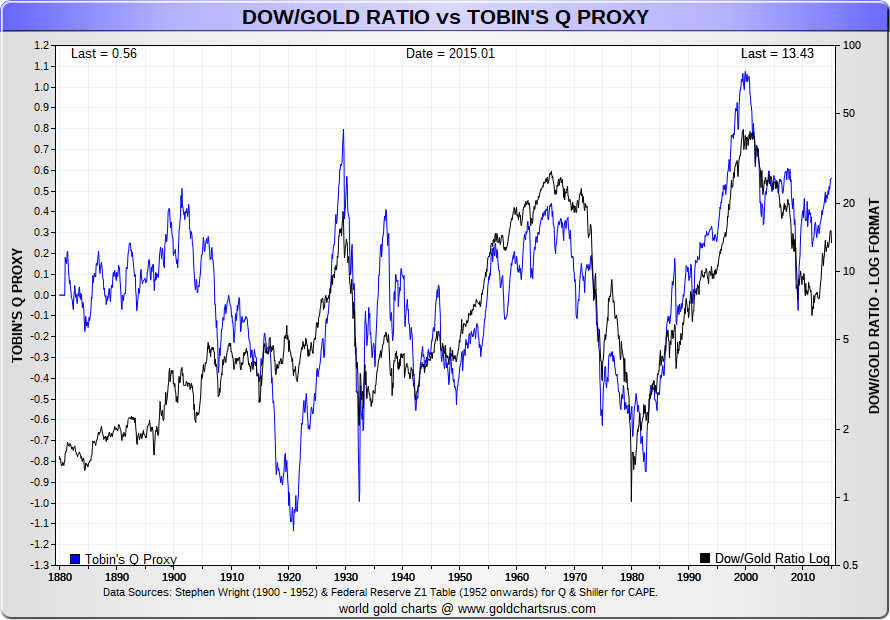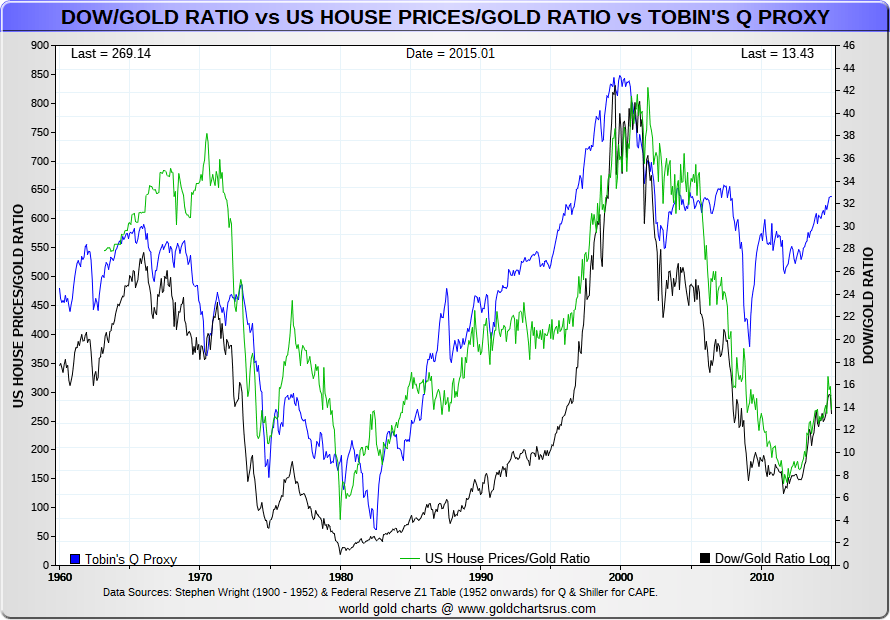Dow / Gold Ratio vs Tobin's Q vs Average US House / Gold Ratio

Back to Free Charts

Dow/Gold Ratio vs Tobin's Q
vs Average US House/Gold Ratio

Data Sources - Tobin's Q - US Ave House PricesA ratio devised by James Tobin of Yale University, Nobel Laureate in Economics, who hypothesized that the combined market value of all the companies on the stock market should be about equal to their replacement costs.
The Q ratio is calculated as the market value of a firm's assets divided by the replacement value of the firm's assets.

For example, a low Q (between 0 and 1) means that the cost to replace a firm's assets is greater than the value of its stock, which implies that the stock is undervalued.
Conversely, a high Q (greater than 1) implies that a firm's stock is relatively more expensive than the replacement cost of its assets, which implies that the stock is overvalued.
This ratio, a measure of stock valuation, is the driving factor behind investment decisions in Tobin's model.
SOURCELinear FormatLog FormatDow/Houses/Gold/TobinsQSite design & maintenance by Nick Laird
All pages on this website are ©1998-2019 Gold Charts R Us - All Rights Reserved